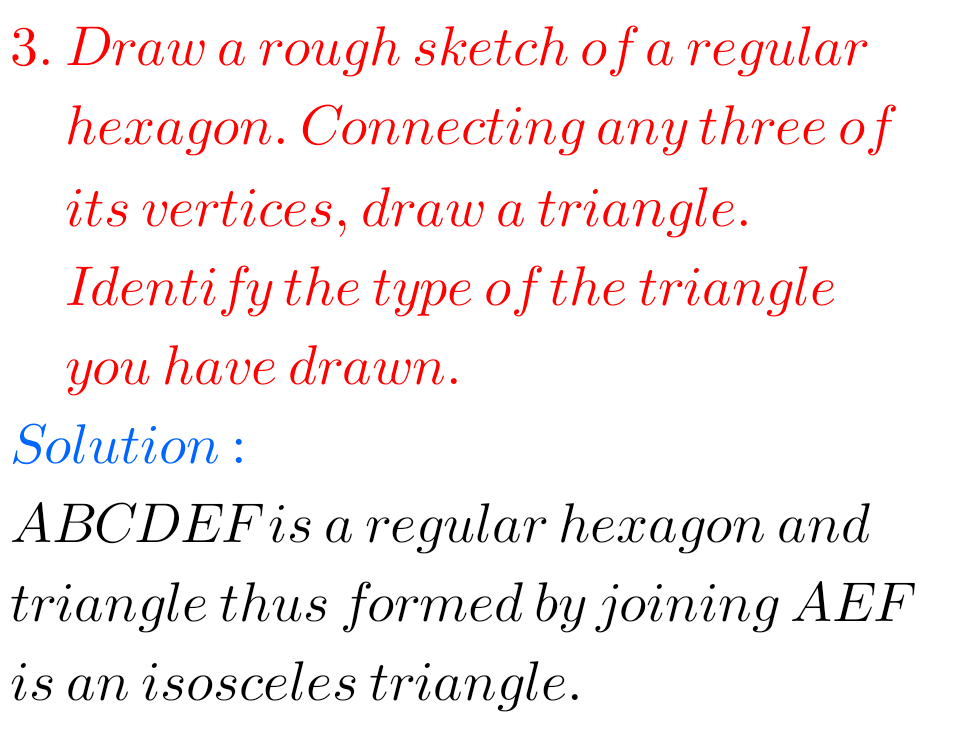## Understanding Elementary Shapes Chapter 5 solutions for exercise 5.8 Ncert Maths class 6

Ncert Maths solutions for Exercise 5.8 class 6 chapter 5 Understanding Elementary Shapes Ncert Maths Class 6 chapter 5 Understanding Elementary Shapes Exercise 5.8 solutions are given. Study the textbook lesson Understanding Elementary Shapes very well. Observe the example problems and solutions given in the textbook. Observe the given solutons and try them in your …